# Two 8-kg blocks A and B resting on shelves are connected by a rod of negligible mass. Knowing that the magnitude of a horizontal force P applied at C is slowly increased from zero, determine the value of P for which motion occurs, and what that motion is, when the coefficient of static friction between all surfaces is (a) µs = 0.40, (b) µs = 0.50.

Question-AnswerCategory: Engineering MechanicsTwo 8-kg blocks A and B resting on shelves are connected by a rod of negligible mass. Knowing that the magnitude of a horizontal force P applied at C is slowly increased from zero, determine the value of P for which motion occurs, and what that motion is, when the coefficient of static friction between all surfaces is (a) µs = 0.40, (b) µs = 0.50.

Two 8-kg blocks A and B resting on shelves are connected by a rod of negligible mass. Knowing that the magnitude of a horizontal force P applied at C is slowly increased from zero, determine the value of P for which motion occurs, and what that motion is, when the coefficient of static friction between all surfaces is (a) µs = 0.40, (b) µs = 0.50.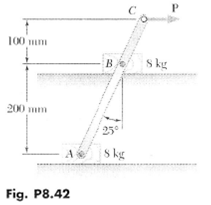Step: 1

Draw the free body diagram of the system.Step: 2

a)
Calculate the friction force at block A,Here, coefficient of static friction is, normal reaction at block A is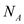Substitute, 0.4 forCalculate the friction force at block B,Here, normal reaction at block B isSubstitute, 0.4 for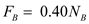Calculate the weight of the block,Step: 3

The equilibrium condition of forces in y-directionTake the condition of equilibrium
Substitute,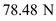for WStep: 4

The equilibrium condition of forces in x-directionSubstitute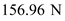for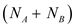Step: 5

Take the moment about the point B
Assume that the moment in the anticlockwise direction is positive.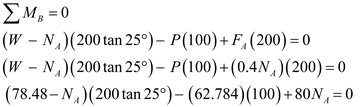So,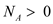Therefore, the system slides
The load P,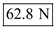b)
Calculate the friction force at block A,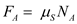Here, coefficient of static friction is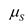, normal reaction at block A isSubstitute, 0.5 for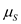Calculate the friction force at block B,Here, normal reaction at block B isSubstitute, 0.5 forStep: 6

The equilibrium condition of forces in x-directionSubstitutefor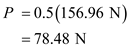Step: 7

Take the moment about the point B
Assume that the moment in the anticlockwise direction is positive.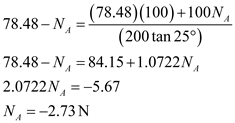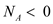, there will be uplift and rotation about B.

Step: 8

For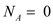, calculate the value of P.
Take the moment about the point B
Assume that the moment in the anticlockwise direction is positive.Therefore, the system rotates about B and the load, P is